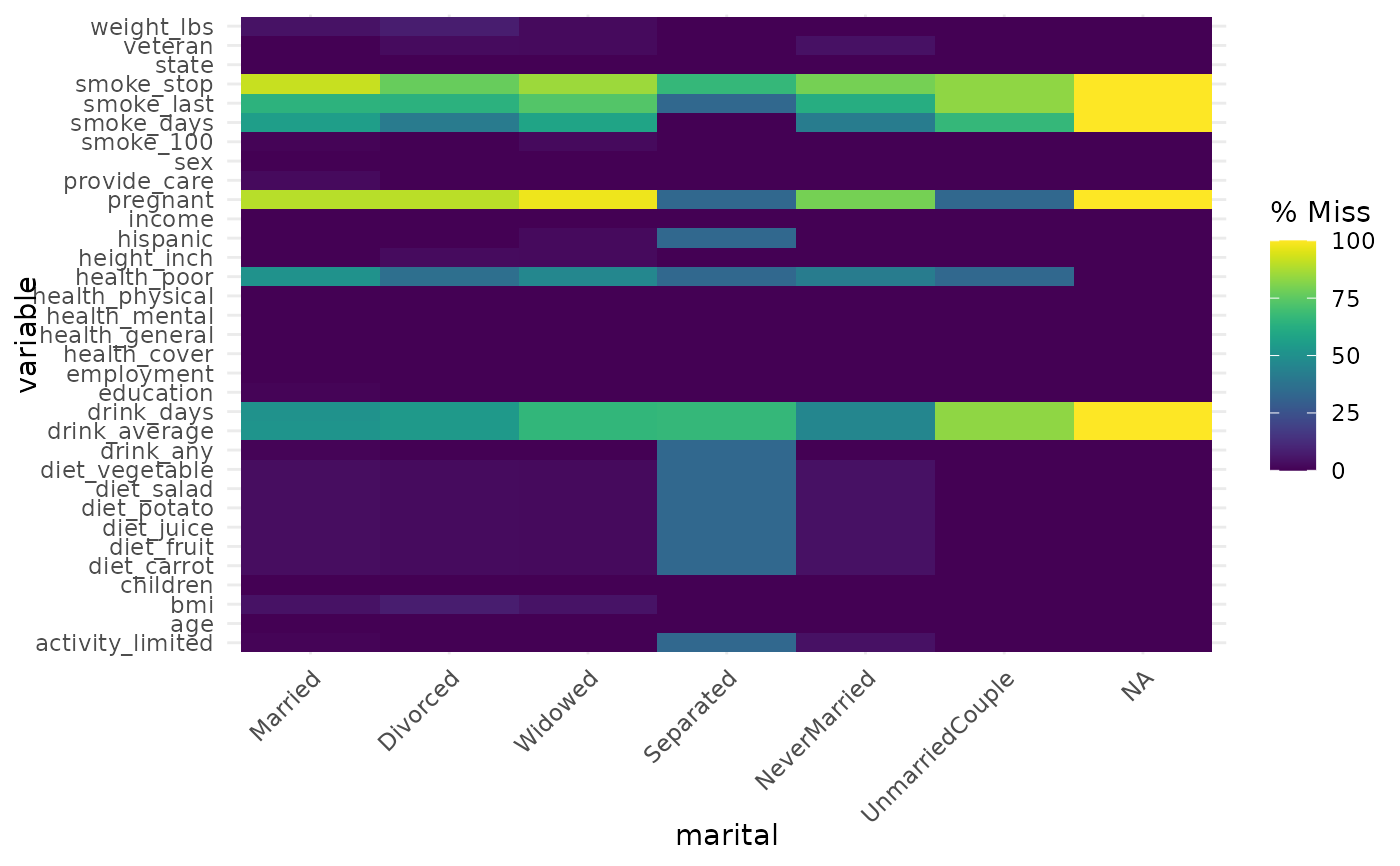This function draws a ggplot plot of the number of missings in each column, broken down by a categorical variable from the dataset. A default minimal theme is used, which can be customised as normal for ggplot.

## Usage

gg_miss_fct(x, fct)

## Arguments

x

data.frame

fct

column containing the factor variable to visualise

## Value

ggplot object depicting the % missing of each factor level for each variable.

geom_miss_point() gg_miss_case() gg_miss_case_cumsum gg_miss_span() gg_miss_var() gg_miss_var_cumsum() gg_miss_which()

## Examples


gg_miss_fct(x = riskfactors, fct = marital)if (FALSE) {
library(ggplot2)
gg_miss_fct(x = riskfactors, fct = marital) + labs(title = "NA in Risk Factors and Marital status")
}